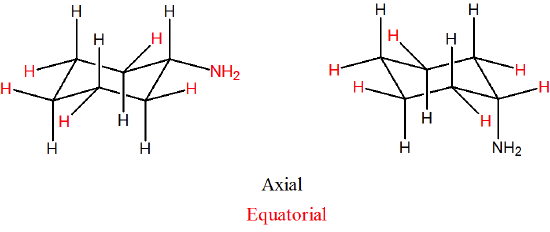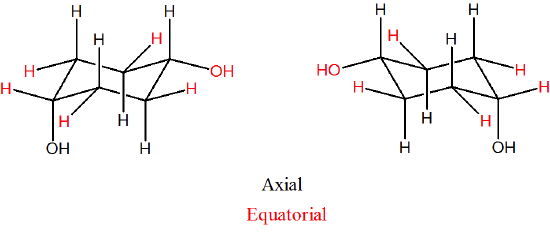# 2.14: Axial and Equatorial Bonds in Cyclohexane

$$\newcommand{\vecs}{\overset { \rightharpoonup} {\mathbf{#1}} }$$ $$\newcommand{\vecd}{\overset{-\!-\!\rightharpoonup}{\vphantom{a}\smash {#1}}}$$$$\newcommand{\id}{\mathrm{id}}$$ $$\newcommand{\Span}{\mathrm{span}}$$ $$\newcommand{\kernel}{\mathrm{null}\,}$$ $$\newcommand{\range}{\mathrm{range}\,}$$ $$\newcommand{\RealPart}{\mathrm{Re}}$$ $$\newcommand{\ImaginaryPart}{\mathrm{Im}}$$ $$\newcommand{\Argument}{\mathrm{Arg}}$$ $$\newcommand{\norm}{\| #1 \|}$$ $$\newcommand{\inner}{\langle #1, #2 \rangle}$$ $$\newcommand{\Span}{\mathrm{span}}$$ $$\newcommand{\id}{\mathrm{id}}$$ $$\newcommand{\Span}{\mathrm{span}}$$ $$\newcommand{\kernel}{\mathrm{null}\,}$$ $$\newcommand{\range}{\mathrm{range}\,}$$ $$\newcommand{\RealPart}{\mathrm{Re}}$$ $$\newcommand{\ImaginaryPart}{\mathrm{Im}}$$ $$\newcommand{\Argument}{\mathrm{Arg}}$$ $$\newcommand{\norm}{\| #1 \|}$$ $$\newcommand{\inner}{\langle #1, #2 \rangle}$$ $$\newcommand{\Span}{\mathrm{span}}$$$$\newcommand{\AA}{\unicode[.8,0]{x212B}}$$

##### Objectives

After completing this section, you should be able to

1. Draw the chair conformation of cyclohexane, with axial and equatorial hydrogen atoms clearly shown and identified.
2. identify the axial and equatorial hydrogens in a given sketch of the cyclohexane molecule.
3. explain how chair conformations of cyclohexane and its derivatives can interconvert through the process of ring flip.
##### Key Terms

Make certain that you can define, and use in context, the key terms below.

• axial position
• equatorial position
• ring flip

## Axial and Equatorial Positions in Cyclohexane

Careful examination of the chair conformation of cyclohexane, shows that the twelve hydrogens are not structurally equivalent. Six of them are located about the periphery of the carbon ring, and are termed equatorial. The other six are oriented above and below the approximate plane of the ring (three in each location), and are termed axial because they are aligned parallel to the symmetry axis of the ring.In the figure above, the equatorial hydrogens are colored blue, and the axial hydrogens are black. Since there are two equivalent chair conformations of cyclohexane in rapid equilibrium, all twelve hydrogens have 50% equatorial and 50% axial character.

### How To Draw Axial and Equatorial BondsHow not to draw the chair:Aside from drawing the basic chair, the key points are:

• Axial bonds alternate up and down, and are shown "vertical".
• Equatorial groups are approximately horizontal, but actually somewhat distorted from that (slightly up or slightly down), so that the angle from the axial group is a bit more than a right angle -- reflecting the common 109.5o bond angle.
• Each carbon has an axial and an equatorial bond.
• Each face of the cyclohexane ring has three axial and three equatorial bonds.
• Each face alternates between axial and equatorial bonds. Then looking at the "up" bond on each carbon in the cyclohexane ring they will alternate axial-equatorial-axial ect.
• When looking down at a cyclohexane ring:
• the equatorial bonds will form an "equator" around the ring.
• The axial bonds will either face towards you or away. These will alternate with each axial bond. The first axial bond will be coming towards with the next going away. There will be three of each type.
• Note! The terms cis and trans in regards to the stereochemistry of a ring are not directly linked to the terms axial and equatorial. It is very common to confuse the two. It typically best not to try and directly inter convert the two naming systems.

### Axial vs. Equatorial Substituents

When a substituent is added to cyclohexane, the ring flip allows for two distinctly different conformations. One will have the substituent in the axial position while the other will have the substituent in the equatorial position. In the next section will discuss the energy differences between these two possible conformations. Below are the two possible chair conformations of methylcyclohexane created by a ring-flip. Although the conformation which places the methyl group in the equatorial position is more stable by 7 kJ/mol, the energy provided by ambient temperature allows the two conformations to rapidly interconvert.The figure below illustrates how to convert a molecular model of cyclohexane between two different chair conformations - this is something that you should practice with models. Notice that a 'ring flip' causes equatorial groups to become axial, and vice-versa.##### Example $$\PageIndex{1}$$

For the following please indicate if the substituents are in the axial or equatorial positions.###### Solution

Due to the large number of bonds in cyclohexane it is common to only draw in the relevant ones (leaving off the hydrogens unless they are involved in a reaction or are important for analysis). It is still possible to determine axial and equatorial positioning with some thought. With problems such as this it is important to remember that each carbon in a cyclohexane ring has one axial and one equatorial bond. Also, remember that axial bonds are perpendicular with the ring and appear to be going either straight up or straight down. Equatorial bonds will be roughly in the plane of the cyclohexane ring (only slightly up or down). Sometimes it is valuable to draw in the additional bonds on the carbons of interest.With this it can be concluded that the bromine and chlorine substituents are attached in equatorial positions and the CH3 substituent is attached in an axial position.

## Exercises

1) Draw two conformations of cyclohexyl amine (C6H11NH2). Indicate axial and equatorial positions.

2) Draw the two isomers of 1,4-dihydroxylcyclohexane, identify which are equatorial and axial.

3) In the following molecule, label which are equatorial and which are axial, then draw the chair flip (showing labels 1,2,3).

## Solutions

1)2)3) Original conformation: 1 = axial, 2 = equatorial, 3 = axial

Flipped chair now looks like this.2.14: Axial and Equatorial Bonds in Cyclohexane is shared under a not declared license and was authored, remixed, and/or curated by LibreTexts.